## P4O10 + 6H20 → 4H3PO4 If 30 g of H2O reacts with 40g of P4O10, what is the limiting reactant? How much H3PO4 can be formed?

Question

P4O10 + 6H20 → 4H3PO4

If 30 g of H2O reacts with 40g of P4O10, what is the limiting reactant? How much H3PO4 can be formed?

in progress 0
5 months 2021-09-05T14:00:46+00:00 1 Answers 3 views 0

## Answers ( )

1. Answer: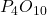is the limiting reagent

66.6 g of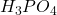will be formed.

Explanation:

To calculate the moles :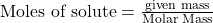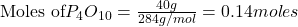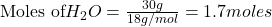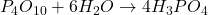According to stoichiometry :

1 mole ofrequire 6 moles of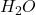Thus 0.14 moles ofwill require=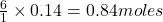ofThusis the limiting reagent as it limits the formation of product andis the excess reagent.

As 1 mole ofgive = 4 moles ofThus 0.17 moles ofgive =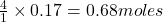ofMass of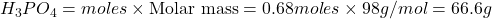Thus 66.6 g ofwill be formed.